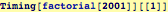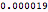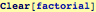Using Recursion to Program

Functions that call themselves...  (It is a good idea to review and review this example until you understand it.

The canonical programming example is the factorial function n! = (n)×(n-1) ×(n-2)×…×(1) where 0! ≡  1; here is a reasonably clever way to use the fact that (n+1)! = (n+1)×n!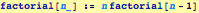try it out...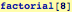Ooops, This isn't what was expected, but upon reflection it is correct--we forgot to define a part of the rule. (Note also that the message window produced an error about recursion limits) Add the second part of the definition. Here, we don't use delayed evaluation (:=) because we want to assign a value immediately.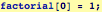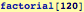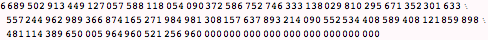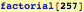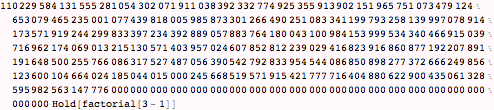Here is where the recursion limit comes in : our function keeps on calling itself (i.e., recursively). Unless a limit is set the program would keep running forever. Mathematica builds in a limit to how many times a function will call itself: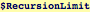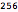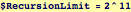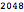Speed versus Memory in Functions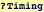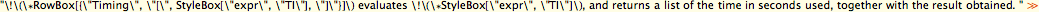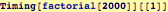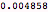Using immediate assignment in a function: spending memory to buy time: Each time the function is called, it makes an extra assignment so that previous values can be recalled if needed.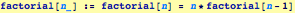This version takes a bit longer the first time, because we are storing data in memory ...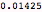But, the next time it is called, the result is much faster.